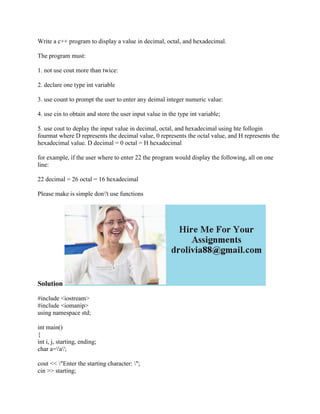Diese Präsentation wurde erfolgreich gemeldet.

# Write a c++ program to display a value in decimal- octal- and hexadeci.docx

Anzeige
Anzeige
Anzeige
Anzeige
Anzeige
Anzeige
Anzeige
Anzeige
Anzeige
Anzeige
Anzeige×

1 von 2 Anzeige

# Write a c++ program to display a value in decimal- octal- and hexadeci.docx

Write a c++ program to display a value in decimal, octal, and hexadecimal.
The program must:
1. not use cout more than twice:
2. declare one type int variable
3. use count to prompt the user to enter any deimal integer numeric value:
4. use cin to obtain and store the user input value in the type int variable;
5. use cout to deplay the input value in decimal, octal, and hexadecimal using hte follogin fourmat where D represents the decimal value, 0 represents the octal value, and H represents the hexadecimal value. D decimal = 0 octal = H hexadecimal
for example, if the user where to enter 22 the program would display the following, all on one line:
22 decimal = 26 octal = 16 hexadecimal
Please make is simple don\'t use functions
Solution
#include <iostream>
#include <iomanip>
using namespace std;
int main()
{
int i, j, starting, ending;
char a=\'a\';
cout << \"Enter the starting character: \";
cin >> starting;
cout << \"CHAR DECIMAL OCTAL HEXADECIMAL\ \";
cout << \"---- ------- ----- ------------\ \";
for (i = 1; i <= starting; i++)
{
cout << \"Enter the ending character: \" << i << endl;
for (j=1; j<=ending; j++)
{
cout << \"\\t \" << int (a) << \" \" << endl;
cout << \"\\t \" << oct << int (a) << \" \" << endl;
cout << \"\\t \" << hex << int (a) << \" \" << endl;
}
}
system (\"PAUSE\");
return 0;
}
.

Write a c++ program to display a value in decimal, octal, and hexadecimal.
The program must:
1. not use cout more than twice:
2. declare one type int variable
3. use count to prompt the user to enter any deimal integer numeric value:
4. use cin to obtain and store the user input value in the type int variable;
5. use cout to deplay the input value in decimal, octal, and hexadecimal using hte follogin fourmat where D represents the decimal value, 0 represents the octal value, and H represents the hexadecimal value. D decimal = 0 octal = H hexadecimal
for example, if the user where to enter 22 the program would display the following, all on one line:
22 decimal = 26 octal = 16 hexadecimal
Please make is simple don\'t use functions
Solution
#include <iostream>
#include <iomanip>
using namespace std;
int main()
{
int i, j, starting, ending;
char a=\'a\';
cout << \"Enter the starting character: \";
cin >> starting;
cout << \"CHAR DECIMAL OCTAL HEXADECIMAL\ \";
cout << \"---- ------- ----- ------------\ \";
for (i = 1; i <= starting; i++)
{
cout << \"Enter the ending character: \" << i << endl;
for (j=1; j<=ending; j++)
{
cout << \"\\t \" << int (a) << \" \" << endl;
cout << \"\\t \" << oct << int (a) << \" \" << endl;
cout << \"\\t \" << hex << int (a) << \" \" << endl;
}
}
system (\"PAUSE\");
return 0;
}
.

Anzeige
Anzeige

### Write a c++ program to display a value in decimal- octal- and hexadeci.docx

1. 1. Write a c++ program to display a value in decimal, octal, and hexadecimal. The program must: 1. not use cout more than twice: 2. declare one type int variable 3. use count to prompt the user to enter any deimal integer numeric value: 4. use cin to obtain and store the user input value in the type int variable; 5. use cout to deplay the input value in decimal, octal, and hexadecimal using hte follogin fourmat where D represents the decimal value, 0 represents the octal value, and H represents the hexadecimal value. D decimal = 0 octal = H hexadecimal for example, if the user where to enter 22 the program would display the following, all on one line: 22 decimal = 26 octal = 16 hexadecimal Please make is simple don't use functions Solution #include <iostream> #include <iomanip> using namespace std; int main() { int i, j, starting, ending; char a='a'; cout << "Enter the starting character: "; cin >> starting;
2. 2. cout << "CHAR DECIMAL OCTAL HEXADECIMAL "; cout << "---- ------- ----- ------------ "; for (i = 1; i <= starting; i++) { cout << "Enter the ending character: " << i << endl; for (j=1; j<=ending; j++) { cout << "t " << int (a) << " " << endl; cout << "t " << oct << int (a) << " " << endl; cout << "t " << hex << int (a) << " " << endl; } } system ("PAUSE"); return 0; }